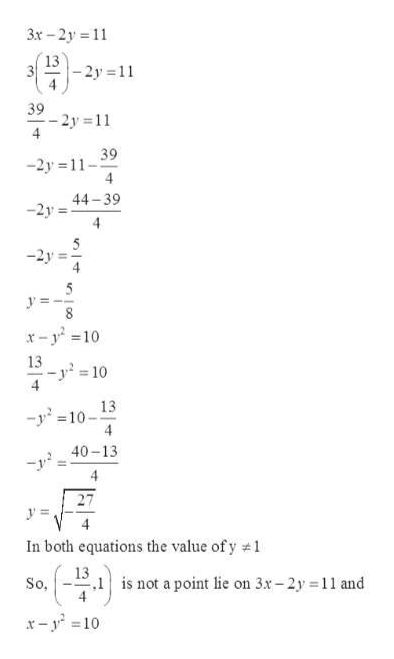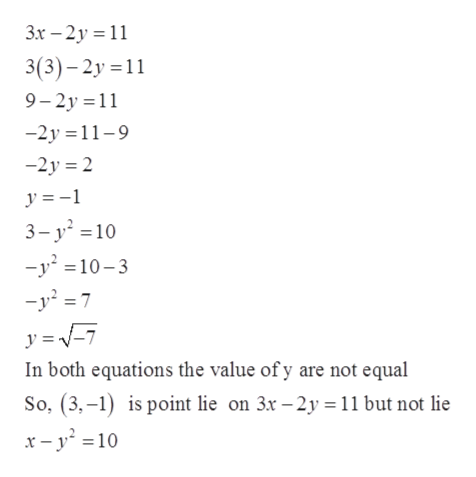# ystem of equations3x-2y=11x-y2 =10pairs given (13/4,1) (3,-1) (5,2) and (0,5/4). The solution(s) of the given system of equations is/are the ordered pair(s)

Question
14 views

ystem of equations

3x-2y=11

x-y=10

pairs given (13/4,1) (3,-1) (5,2) and (0,5/4).

The solution(s) of the given system of equations is/are the ordered pair(s)

check_circle

Step 1

Given:

Step 2

Check for (13/4, 1):

Here, x = 13/4

So,help_outlineImage Transcriptionclose3x-2y 11 13 -2y 11 39 2y 11 4 39 -2y 11 4 44-39 -2y 4 -2y 5 У x-y10 13 -y2 4 = 10 13 -y210 4 40-13 уз In both equations the value ofy 1 13 ,1is not a point lie on 3x-2y 11 and So, x-y10 fullscreen
Step 3

Similarly, check for (3, - 1):

H...help_outlineImage Transcriptionclose3x-2y 11 3(3)-2y 1 9-2y 11 -2y 11-9 -2y 2 y =1 3- y2 10 -y210-3 -y27 y In both equations the value of y are not equal So, (3,-1) is point lie on 3x -2y 11 but not lie x -y10 fullscreen

### Want to see the full answer?

See Solution

#### Want to see this answer and more?

Solutions are written by subject experts who are available 24/7. Questions are typically answered within 1 hour.*

See Solution
*Response times may vary by subject and question.
Tagged in

### Other